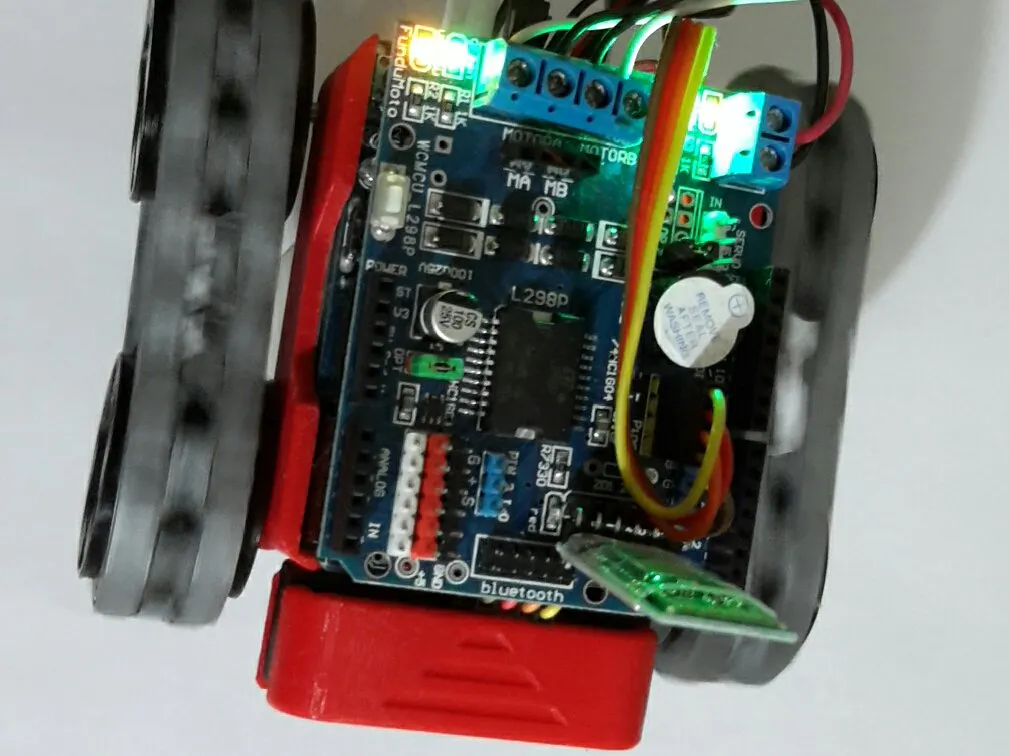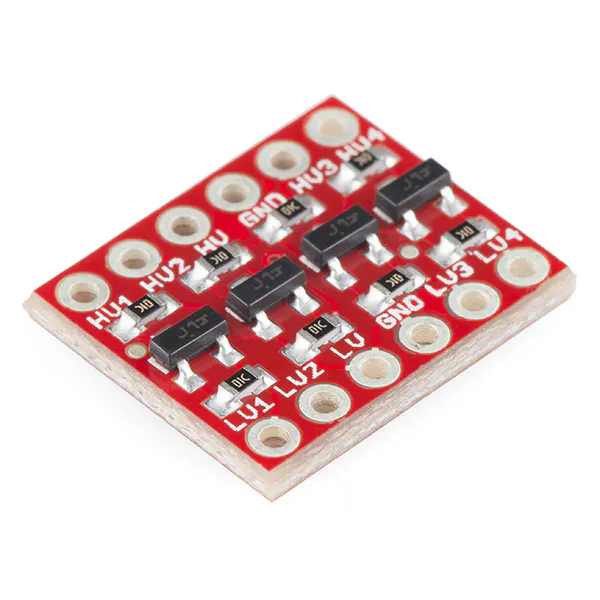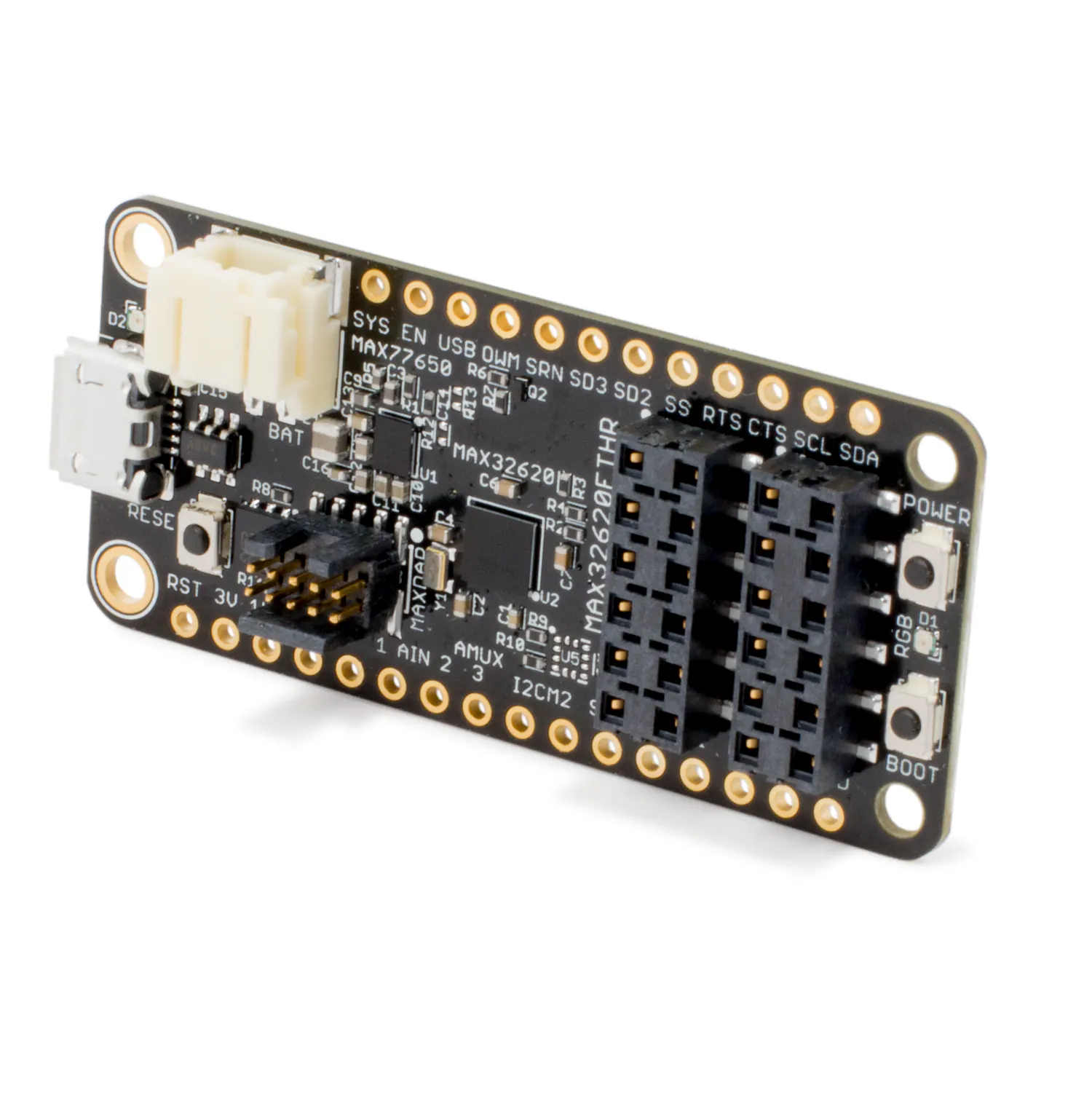Flexible Robotic Car Based on MAX32620FTHR

This project demonstrates the use of MAX32620FTHR for replacement of Arduino in a smart robot car.

IntermediateFull instructions provided2 days400Things used in this project

Hardware componentsSparkFun Level Shifter Board
×1Maxim Integrated MAX32620FTHR
×1
 fundumoto
×1

Software apps and online servicesArduino IDE

Hand tools and fabrication machinesSoldering iron (generic)

Code

discharge test log

Plain text
A charge and discharge test on the 2000 mAh battery
Set Main Bias to normal Mode: okay
Set On/Off-Button to push-button-mode: okay
Set nEN input debounce time to 30ms: okay
Comparing part-numbers: okay
Checking OTP options: okay
Set the VCOLD JEITA Temperature Threshold to 0C: okay
Set the VCOOL JEITA Temperature Threshold to 15C: okay
Set the VWARM JEITA Temperature Threshold to 45C: okay
Set the VHOT JEITA Temperature Threshold to 60C: okay
Set CHGIN regulation voltage to 4.00V: okay
Set CHGIN Input Current Limit to 300mA: okay
Set the prequalification charge current to 10%: okay
Set Battery prequalification voltage threshold to 3.0V: okay
Set Charger Termination Current to 15% of of fast charge current: okay
Set Topoff timer value to 0 minutes: okay
Set the die junction temperature regulation point to 60C: okay
Set System voltage regulation to 4.50V: okay
Set the fast-charge constant current value to 300mA: okay
Set the fast-charge safety timer to 3h: okay
Set IFAST-CHG_JEITA to 300mA: okay
Set Thermistor enable bit: okay
Set fast-charge battery regulation voltage to 4.20V: okay
Set USB not in power down: okay
Set the modified VFAST-CHG to 4.00V: okay
Selects the battery discharge current full-scale current value to 300mA: okay
Disable the analog MUX output: okay
Set the Charger to Enable: okay
Disable SIMO Buck-Boost Channel 0 Active-Discharge: okay
Disable SIMO Buck-Boost Channel 1 Active-Discharge: okay
Disable SIMO Buck-Boost Channel 2 Active-Discharge: okay
Set SIMO Buck-Boost to maximum drive strength: okay
Set SIMO Buck-Boost Channel 0 Peak Current Limit to 500mA: okay
Set SIMO Buck-Boost Channel 1 Peak Current Limit to 500mA: okay
Set SIMO Buck-Boost Channel 2 Peak Current Limit to 500mA: okay
Set SIMO Buck-Boost Channel 2 to on while in stand-by-mode: okay
Initialize Global Interrupt Mask Register: okay
Initialize Charger Interrupt Mask Register: okay
End Initialisation of MAX77650
Capacity of plugged in battery is: 1791.0000 mAH

State of Charge value is: %89.5625

Setup Done
****** Battery Telemetry ******
Get Reported Capacity
Capacity of plugged in battery is: 1792.0000 mAH

Get Resist Sensor
Resist sensor value is: 0.0500 ohm

Get State of Charge
State of Charge value is: %89.5977

Get instantaneous voltage
Instantaneous Voltage is: 4.1177 V

Get instantaneous current
Instantaneous Current is: 286.0625 mA

Get Average current
Average Current is: 29.3438 mA

Get time to empty
Time to empty is: 102.3984 Hours

****** Battery Telemetry ******
Get Reported Capacity
Capacity of plugged in battery is: 1795.0000 mAH

Get Resist Sensor
Resist sensor value is: 0.0500 ohm

Get State of Charge
State of Charge value is: %89.7930

Get instantaneous voltage
Instantaneous Voltage is: 4.1198 V

Get instantaneous current
Instantaneous Current is: 291.2500 mA

Get Average current
Average Current is: 122.6563 mA

Get time to empty
Time to empty is: 102.3984 Hours

****** Battery Telemetry ******
Get Reported Capacity
Capacity of plugged in battery is: 1799.0000 mAH

Get Resist Sensor
Resist sensor value is: 0.0500 ohm

Get State of Charge
State of Charge value is: %89.9883

Get instantaneous voltage
Instantaneous Voltage is: 4.1220 V

Get instantaneous current
Instantaneous Current is: 296.8750 mA

Get Average current
Average Current is: 185.8750 mA

Get time to empty
Time to empty is: 102.3984 Hours

****** Battery Telemetry ******
Get Reported Capacity
Capacity of plugged in battery is: 1803.0000 mAH

Get Resist Sensor
Resist sensor value is: 0.0500 ohm

Get State of Charge
State of Charge value is: %90.1953

Get instantaneous voltage
Instantaneous Voltage is: 4.1222 V

Get instantaneous current
Instantaneous Current is: 298.0000 mA

Get Average current
Average Current is: 225.0937 mA

Get time to empty
Time to empty is: 102.3984 Hours

****** Battery Telemetry ******
Get Reported Capacity
Capacity of plugged in battery is: 1807.0000 mAH

Get Resist Sensor
Resist sensor value is: 0.0500 ohm

Get State of Charge
State of Charge value is: %90.3906

Get instantaneous voltage
Instantaneous Voltage is: 4.1236 V

Get instantaneous current
Instantaneous Current is: 297.9062 mA

Get Average current
Average Current is: 250.6250 mA

Get time to empty
Time to empty is: 102.3984 Hours

****** Battery Telemetry ******
Get Reported Capacity
Capacity of plugged in battery is: 1811.0000 mAH

Get Resist Sensor
Resist sensor value is: 0.0500 ohm

Get State of Charge
State of Charge value is: %90.5859

Get instantaneous voltage
Instantaneous Voltage is: 4.1216 V

Get instantaneous current
Instantaneous Current is: 289.7812 mA

Get Average current
Average Current is: 265.7812 mA

Get time to empty
Time to empty is: 102.3984 Hours

****** Battery Telemetry ******
Get Reported Capacity
Capacity of plugged in battery is: 1815.0000 mAH

Get Resist Sensor
Resist sensor value is: 0.0500 ohm

Get State of Charge
State of Charge value is: %90.7813

Get instantaneous voltage
Instantaneous Voltage is: 4.1253 V

Get instantaneous current
Instantaneous Current is: 288.6250 mA

Get Average current
Average Current is: 275.3750 mA

Get time to empty
Time to empty is: 102.3984 Hours

****** Battery Telemetry ******
Get Reported Capacity
Capacity of plugged in battery is: 1819.0000 mAH

Get Resist Sensor
Resist sensor value is: 0.0500 ohm

Get State of Charge
State of Charge value is: %90.9883

Get instantaneous voltage
Instantaneous Voltage is: 4.1291 V

Get instantaneous current
Instantaneous Current is: 293.5000 mA

Get Average current
Average Current is: 282.9375 mA

Get time to empty
Time to empty is: 102.3984 Hours

****** Battery Telemetry ******
Get Reported Capacity
Capacity of plugged in battery is: 1823.0000 mAH

Get Resist Sensor
Resist sensor value is: 0.0500 ohm

Get State of Charge
State of Charge value is: %91.1836

Get instantaneous voltage
Instantaneous Voltage is: 4.1352 V

Get instantaneous current
Instantaneous Current is: 292.3125 mA

Get Average current
Average Current is: 287.0000 mA

Get time to empty
Time to empty is: 102.3984 Hours

****** Battery Telemetry ******
Get Reported Capacity
Capacity of plugged in battery is: 1827.0000 mAH

Get Resist Sensor
Resist sensor value is: 0.0500 ohm

Get State of Charge
State of Charge value is: %91.3789

Get instantaneous voltage
Instantaneous Voltage is: 4.1336 V

Get instantaneous current
Instantaneous Current is: 290.9687 mA

Get Average current
Average Current is: 289.0937 mA

Get time to empty
Time to empty is: 102.3984 Hours

****** Battery Telemetry ******
Get Reported Capacity
Capacity of plugged in battery is: 1831.0000 mAH

Get Resist Sensor
Resist sensor value is: 0.0500 ohm

Get State of Charge
State of Charge value is: %91.5742

Get instantaneous voltage
Instantaneous Voltage is: 4.1325 V

Get instantaneous current
Instantaneous Current is: 282.4375 mA

Get Average current
Average Current is: 290.6250 mA

Get time to empty
Time to empty is: 102.3984 Hours

****** Battery Telemetry ******
Get Reported Capacity
Capacity of plugged in battery is: 1835.0000 mAH

Get Resist Sensor
Resist sensor value is: 0.0500 ohm

Get State of Charge
State of Charge value is: %91.7813

Get instantaneous voltage
Instantaneous Voltage is: 4.1352 V

Get instantaneous current
Instantaneous Current is: 292.8125 mA

Get Average current
Average Current is: 292.1562 mA

Get time to empty
Time to empty is: 102.3984 Hours

****** Battery Telemetry ******
Get Reported Capacity
Capacity of plugged in battery is: 1839.0000 mAH

Get Resist Sensor
Resist sensor value is: 0.0500 ohm

Get State of Charge
State of Charge value is: %91.9648

Get instantaneous voltage
Instantaneous Voltage is: 4.1356 V

Get instantaneous current
Instantaneous Current is: 293.5000 mA

Get Average current
Average Current is: 292.6250 mA

Get time to empty
Time to empty is: 102.3984 Hours

****** Battery Telemetry ******
Get Reported Capacity
Capacity of plugged in battery is: 1843.0000 mAH

Get Resist Sensor
Resist sensor value is: 0.0500 ohm

Get State of Charge
State of Charge value is: %92.1719

Get instantaneous voltage
Instantaneous Voltage is: 4.1328 V

Get instantaneous current
Instantaneous Current is: 285.7187 mA

Get Average current
Average Current is: 292.4375 mA

Get time to empty
Time to empty is: 102.3984 Hours

****** Battery Telemetry ******
Get Reported Capacity
Capacity of plugged in battery is: 1847.0000 mAH

Get Resist Sensor
Resist sensor value is: 0.0500 ohm

Get State of Charge
State of Charge value is: %92.3555

Get instantaneous voltage
Instantaneous Voltage is: 4.1366 V

Get instantaneous current
Instantaneous Current is: 297.2500 mA

Get Average current
Average Current is: 292.9375 mA

Get time to empty
Time to empty is: 102.3984 Hours

****** Battery Telemetry ******
Get Reported Capacity
Capacity of plugged in battery is: 1851.0000 mAH

Get Resist Sensor
Resist sensor value is: 0.0500 ohm

Get State of Charge
State of Charge value is: %92.5625

Get instantaneous voltage
Instantaneous Voltage is: 4.1278 V

Get instantaneous current
Instantaneous Current is: 289.6250 mA

Get Average current
Average Current is: 293.2500 mA

Get time to empty
Time to empty is: 102.3984 Hours

****** Battery Telemetry ******
Get Reported Capacity
Capacity of plugged in battery is: 1855.0000 mAH

Get Resist Sensor
Resist sensor value is: 0.0500 ohm

Get State of Charge
State of Charge value is: %92.7461

Get instantaneous voltage
Instantaneous Voltage is: 4.1388 V

Get instantaneous current
Instantaneous Current is: 292.9375 mA

Get Average current
Average Current is: 293.5625 mA

Get time to empty
Time to empty is: 102.3984 Hours

****** Battery Telemetry ******
Get Reported Capacity
Capacity of plugged in battery is: 1858.0000 mAH

Get Resist Sensor
Resist sensor value is: 0.0500 ohm

Get State of Charge
State of Charge value is: %92.9414

Get instantaneous voltage
Instantaneous Voltage is: 4.1406 V

Get instantaneous current
Instantaneous Current is: 292.1250 mA

Get Average current
Average Current is: 293.5625 mA

Get time to empty
Time to empty is: 102.3984 Hours

****** Battery Telemetry ******
Get Reported Capacity
Capacity of plugged in battery is: 1862.0000 mAH

Get Resist Sensor
Resist sensor value is: 0.0500 ohm

Get State of Charge
State of Charge value is: %93.1367

Get instantaneous voltage
Instantaneous Voltage is: 4.1392 V

Get instantaneous current
Instantaneous Current is: 297.8125 mA

Get Average current
Average Current is: 293.3437 mA

Get time to empty
Time to empty is: 102.3984 Hours

****** Battery Telemetry ******
Get Reported Capacity
Capacity of plugged in battery is: 1866.0000 mAH

Get Resist Sensor
Resist sensor value is: 0.0500 ohm

Get State of Charge
State of Charge value is: %93.3320

Get instantaneous voltage
Instantaneous Voltage is: 4.1386 V

Get instantaneous current
Instantaneous Current is: 298.0937 mA

Get Average current
Average Current is: 293.8437 mA

Get time to empty
Time to empty is: 102.3984 Hours

****** Battery Telemetry ******
Get Reported Capacity
Capacity of plugged in battery is: 1870.0000 mAH

Get Resist Sensor
Resist sensor value is: 0.0500 ohm

Get State of Charge
State of Charge value is: %93.5156

Get instantaneous voltage
Instantaneous Voltage is: 4.1405 V

Get instantaneous current
Instantaneous Current is: 291.3125 mA

Get Average current
Average Current is: 293.9687 mA

Get time to empty
Time to empty is: 102.3984 Hours

****** Battery Telemetry ******
Get Reported Capacity
Capacity of plugged in battery is: 1874.0000 mAH

Get Resist Sensor
Resist sensor value is: 0.0500 ohm

Get State of Charge
State of Charge value is: %93.7109

Get instantaneous voltage
Instantaneous Voltage is: 4.1436 V

Get instantaneous current
Instantaneous Current is: 291.9062 mA

Get Average current
Average Current is: 293.9062 mA

Get time to empty
Time to empty is: 102.3984 Hours

****** Battery Telemetry ******
Get Reported Capacity
Capacity of plugged in battery is: 1878.0000 mAH

Get Resist Sensor
Resist sensor value is: 0.0500 ohm

Get State of Charge
State of Charge value is: %93.9063

Get instantaneous voltage
Instantaneous Voltage is: 4.1422 V

Get instantaneous current
Instantaneous Current is: 300.9062 mA

Get Average current
Average Current is: 293.7812 mA

Get time to empty
Time to empty is: 102.3984 Hours

****** Battery Telemetry ******
Get Reported Capacity
Capacity of plugged in battery is: 1881.0000 mAH

Get Resist Sensor
Resist sensor value is: 0.0500 ohm

Get State of Charge
State of Charge value is: %94.1016

Get instantaneous voltage
Instantaneous Voltage is: 4.1409 V

Get instantaneous current
Instantaneous Current is: 290.6250 mA

Get Average current
Average Current is: 293.9062 mA

Get time to empty
Time to empty is: 102.3984 Hours

****** Battery Telemetry ******
Get Reported Capacity
Capacity of plugged in battery is: 1885.0000 mAH

Get Resist Sensor
Resist sensor value is: 0.0500 ohm

Get State of Charge
State of Charge value is: %94.2969

Get instantaneous voltage
Instantaneous Voltage is: 4.1437 V

Get instantaneous current
Instantaneous Current is: 292.4062 mA

Get Average current
Average Current is: 293.9062 mA

Get time to empty
Time to empty is: 102.3984 Hours

****** Battery Telemetry ******
Get Reported Capacity
Capacity of plugged in battery is: 1889.0000 mAH

Get Resist Sensor
Resist sensor value is: 0.0500 ohm

Get State of Charge
State of Charge value is: %94.4805

Get instantaneous voltage
Instantaneous Voltage is: 4.1425 V

Get instantaneous current
Instantaneous Current is: 294.4062 mA

Get Average current
Average Current is: 293.5937 mA

Get time to empty
Time to empty is: 102.3984 Hours

****** Battery Telemetry ******
Get Reported Capacity
Capacity of plugged in battery is: 1893.0000 mAH

Get Resist Sensor
Resist sensor value is: 0.0500 ohm

Get State of Charge
State of Charge value is: %94.6641

Get instantaneous voltage
Instantaneous Voltage is: 4.1442 V

Get instantaneous current
Instantaneous Current is: 289.3437 mA

Get Average current
Average Current is: 293.0312 mA

Get time to empty
Time to empty is: 102.3984 Hours

****** Battery Telemetry ******
Get Reported Capacity
Capacity of plugged in battery is: 1897.0000 mAH

Get Resist Sensor
Resist sensor value is: 0.0500 ohm

Get State of Charge
State of Charge value is: %94.8594

Get instantaneous voltage
Instantaneous Voltage is: 4.1475 V

Get instantaneous current
Instantaneous Current is: 297.5312 mA

Get Average current
Average Current is: 293.2812 mA

Get time to empty
Time to empty is: 102.3984 Hours

****** Battery Telemetry ******
Get Reported Capacity
Capacity of plugged in battery is: 1900.0000 mAH

Get Resist Sensor
Resist sensor value is: 0.0500 ohm

Get State of Charge
State of Charge value is: %95.0430

Get instantaneous voltage
Instantaneous Voltage is: 4.1448 V

Get instantaneous current
Instantaneous Current is: 295.5625 mA

Get Average current
Average Current is: 292.7500 mA

Get time to empty
Time to empty is: 102.3984 Hours

****** Battery Telemetry ******
Get Reported Capacity
Capacity of plugged in battery is: 1904.0000 mAH

Get Resist Sensor
Resist sensor value is: 0.0500 ohm

Get State of Charge
State of Charge value is: %95.2266

Get instantaneous voltage
Instantaneous Voltage is: 4.1414 V

Get instantaneous current
Instantaneous Current is: 298.7812 mA

Get Average current
Average Current is: 293.2187 mA

Get time to empty
Time to empty is: 102.3984 Hours

****** Battery Telemetry ******
Get Reported Capacity
Capacity of plugged in battery is: 1908.0000 mAH

Get Resist Sensor
Resist sensor value is: 0.0500 ohm

Get State of Charge
State of Charge value is: %95.4219

Get instantaneous voltage
Instantaneous Voltage is: 4.1448 V

Get instantaneous current
Instantaneous Current is: 290.8437 mA

Get Average current
Average Current is: 293.2500 mA

Get time to empty
Time to empty is: 102.3984 Hours

****** Battery Telemetry ******
Get Reported Capacity
Capacity of plugged in battery is: 1912.0000 mAH

Get Resist Sensor
Resist sensor value is: 0.0500 ohm

Get State of Charge
State of Charge value is: %95.6055

Get instantaneous voltage
Instantaneous Voltage is: 4.1462 V

Get instantaneous current
Instantaneous Current is: 298.8437 mA

Get Average current
Average Current is: 293.1250 mA
...

This file has been truncated, please download it to see its full contents.

Smars demo code

Demo code for SMARS

Credits

Manuel Alejandro Iglesias Abbatemarco

10 projects • 48 followers
Embedded Firmware Engineer